Sequences And Series Of Real Numbers -4

# Sequences And Series Of Real Numbers -4

Test Description

## 20 Questions MCQ Test Topic-wise Tests & Solved Examples for IIT JAM Mathematics | Sequences And Series Of Real Numbers -4

Sequences And Series Of Real Numbers -4 for IIT JAM 2023 is part of Topic-wise Tests & Solved Examples for IIT JAM Mathematics preparation. The Sequences And Series Of Real Numbers -4 questions and answers have been prepared according to the IIT JAM exam syllabus.The Sequences And Series Of Real Numbers -4 MCQs are made for IIT JAM 2023 Exam. Find important definitions, questions, notes, meanings, examples, exercises, MCQs and online tests for Sequences And Series Of Real Numbers -4 below.
Solutions of Sequences And Series Of Real Numbers -4 questions in English are available as part of our Topic-wise Tests & Solved Examples for IIT JAM Mathematics for IIT JAM & Sequences And Series Of Real Numbers -4 solutions in Hindi for Topic-wise Tests & Solved Examples for IIT JAM Mathematics course. Download more important topics, notes, lectures and mock test series for IIT JAM Exam by signing up for free. Attempt Sequences And Series Of Real Numbers -4 | 20 questions in 60 minutes | Mock test for IIT JAM preparation | Free important questions MCQ to study Topic-wise Tests & Solved Examples for IIT JAM Mathematics for IIT JAM Exam | Download free PDF with solutions
 1 Crore+ students have signed up on EduRev. Have you?
Sequences And Series Of Real Numbers -4 - Question 1

### If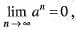then

Detailed Solution for Sequences And Series Of Real Numbers -4 - Question 1

Explanation : For |a|<1

b = 1/a, |b|>1

lim (n→∞) an = lim (n→∞) (1/b)n

= lim(n→∞) 1/bn

= 1/(±∞)

= 0

Sequences And Series Of Real Numbers -4 - Question 2

### Which amongst the following statements is true?

Detailed Solution for Sequences And Series Of Real Numbers -4 - Question 2

Option c will be correct answer of this . every bounded sequence is not convergent for example (-1)^n is bounded by -1 and 1 but it is not convergent .

Sequences And Series Of Real Numbers -4 - Question 3

### A Cauchy sequence is convergent, if it is a

Sequences And Series Of Real Numbers -4 - Question 4

If radius of convergence of series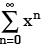is 1, then radius of convergence of series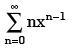is…

Detailed Solution for Sequences And Series Of Real Numbers -4 - Question 4

By the differentiation’s theorem, we have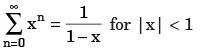...(i)

Differentiating term by term, we obtain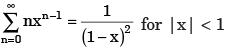...(ii)

So, both the series have same radius of convergence.

Sequences And Series Of Real Numbers -4 - Question 5

If {xn} and {yn} are two convergent sequence such that xn < yn,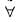n ∈ N, then

Detailed Solution for Sequences And Series Of Real Numbers -4 - Question 5

The correct option is Option A.

Let lim xn = x and lim yn = y and zn = yn - xn

n->∞                n->∞

Then (zn) is a convergent sequence such that zn > 0 ∇ n ∊N

and lim zn = y - x.

n->∞

Now (zn) is a convergent sequence of real numbers and zn > 0 ∇ n ∊N

So, lim zn ≥ 0

n->∞

So, y - x ≥ 0

=> y ≥ x

=> lim yn ≥ lim xn

n->∞      n->∞

Hence, proved.

Sequences And Series Of Real Numbers -4 - Question 6

Let a = min{x2 + 2x + 3, x ∈ R} and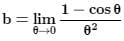then the value of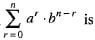Detailed Solution for Sequences And Series Of Real Numbers -4 - Question 6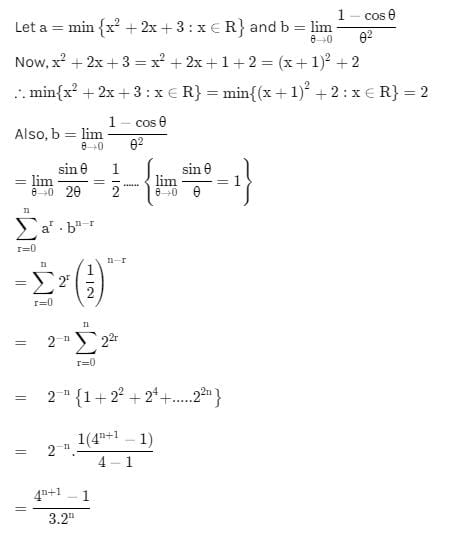Sequences And Series Of Real Numbers -4 - Question 7

The sequence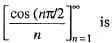Detailed Solution for Sequences And Series Of Real Numbers -4 - Question 7

Explanation : Suppose otherwise, that there exists a number L implies R and a positive integer N such that

| f(n) - L | < e {for all } e > 0 {for all } n > N.

Since N is a positive integer, we know 4N > N and 4N+2 > N.

But,

f(4N) = cos (2N pi) = 1 and f(4N+2) = cos((2N+1)pi) = -1

Taking e = 1/2

= |1 - L| < 1/2 and |-1-L| < 1/2

=> |1+L| < 1/2

But, these imply

|1-L| + |1+L| < 1.

By the triangle inequality we then have

|1 - L + 1 + L | < 1

=> 2 < 1

a contradiction. Hence, there is no such limit L.

Therefore, the sequence converges to zero.

Sequences And Series Of Real Numbers -4 - Question 8

If for any e > 0, there exists a positive integer m such that I an - am | < e whenever n ≥ m . The sequence an > is called

Sequences And Series Of Real Numbers -4 - Question 9

A convergent sequence is a Cauchy sequence, if it is a

Detailed Solution for Sequences And Series Of Real Numbers -4 - Question 9

Solution :- If {an}∞n=1 is a cauchy sequence of real numbers and if there is a sub-sequence of this sequence, {anj}∞j=1 which converges to a real number L, then I need to show that the sequence {an}∞n=1 converges to the real number L.

Sequences And Series Of Real Numbers -4 - Question 10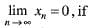Sequences And Series Of Real Numbers -4 - Question 11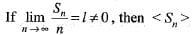Sequences And Series Of Real Numbers -4 - Question 12

For the given Arithmetic progression find the position of first negative term? 50, 47, 44, 41,............

Detailed Solution for Sequences And Series Of Real Numbers -4 - Question 12

Let nth term=0, the next term would be first negative term.
0 = 50 + (n - 1) – 3, n = 17.66.. therefore at n = 18 the first negative term would occur.

Sequences And Series Of Real Numbers -4 - Question 13

In the given AP series the term at position 11 would be? 5, 8, 11, 14, 17, 20.........50.

Detailed Solution for Sequences And Series Of Real Numbers -4 - Question 13

nth term = a + (n – 1)d, nth term = 5 + (11 - 1)3 = 35.

Sequences And Series Of Real Numbers -4 - Question 14

The value of K for which the set of equations x + ky + 3z = 0, 3x + ky - 2z = 0, 2x + 3y - 4z = 0, has a non-trivial solution over the set of rationales is

Detailed Solution for Sequences And Series Of Real Numbers -4 - Question 14

For non-trivial soln det of Coefficient, matrix should be 0.
|1 K 3|
|3 K 2| =  0
|2 3 4|
4K - 4K + 27 - 6K + 6 + 12K = 0
2K + 33 = 0
33 K=2
K=33/2

Sequences And Series Of Real Numbers -4 - Question 15

If sequences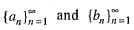are convergent, then

Sequences And Series Of Real Numbers -4 - Question 16

A sequence contains a convergent subsequence, if it is

Sequences And Series Of Real Numbers -4 - Question 17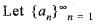be a sequence converges to 0 and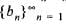be a sequence that is bounded, then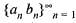is a sequence that

Sequences And Series Of Real Numbers -4 - Question 18

Which of the following sequences of functions is uniformly convergent on (0, 1)?

Detailed Solution for Sequences And Series Of Real Numbers -4 - Question 18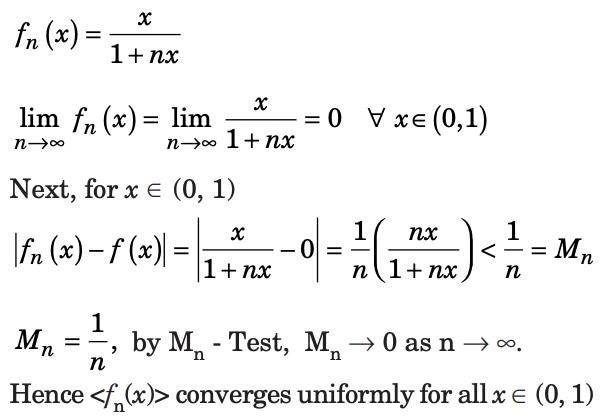Sequences And Series Of Real Numbers -4 - Question 19

The sequence {xn}, where xn = nl/n, converge to

Sequences And Series Of Real Numbers -4 - Question 20

Let xn = 22n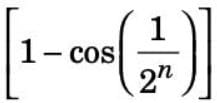for all n ∈ N. Then the sequence {xn}

Detailed Solution for Sequences And Series Of Real Numbers -4 - Question 20

xn = 22n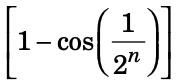for all n ∈ N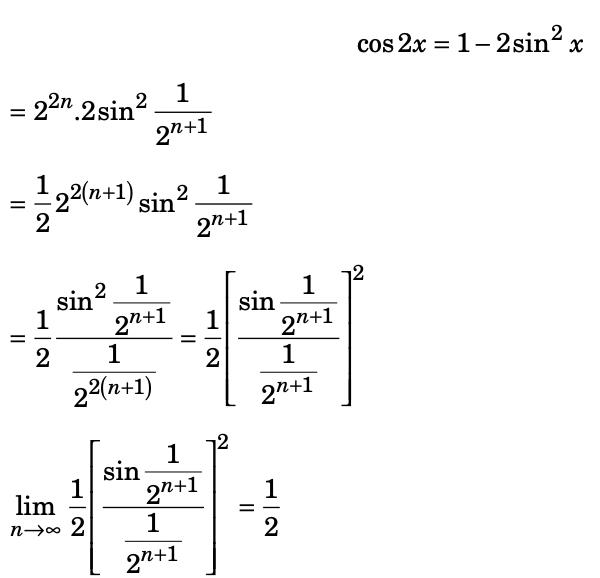## Topic-wise Tests & Solved Examples for IIT JAM Mathematics

27 docs|150 tests
 Use Code STAYHOME200 and get INR 200 additional OFF Use Coupon Code
Information about Sequences And Series Of Real Numbers -4 Page
In this test you can find the Exam questions for Sequences And Series Of Real Numbers -4 solved & explained in the simplest way possible. Besides giving Questions and answers for Sequences And Series Of Real Numbers -4, EduRev gives you an ample number of Online tests for practice

## Topic-wise Tests & Solved Examples for IIT JAM Mathematics

27 docs|150 tests